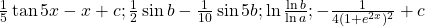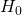# 2021 A-level H2 Mathematics (9758) Paper 2 Suggested SolutionsAll solutions here are suggested. Mr. Teng will hold no liability for any errors. Comments are entirely personal opinions.

Question 1:Question 2:Question 3:Question 4:; away from the starting point
Question 5:Question 6:Question 7:Question 8:Question 9:Question 10:Question 11:•Phua

Wasn’t 8 in thousand miles?

•KS Teng

Yes, Both are ok, it really depends on what youris based on, depends on whether you usedor.

•george

hi for qn 2, what’s the rewritten equation, the one that shows area larger than A? the one mark qn

•KS Teng

Hi, yes, the equation is there. As for other parts, I’ll add it in now. Sorry, due to some issues and complaints, I can’t post so fast.

•jack

hi for qn 8, i used everything in terms of thousand miles, except the first part where i put my hypothesis as 20000. but for part c i changed it to 20. is it ok to do so or if no, how many makrs will i lose?

•Steel

Bro I did the same thing. I think as long as you stated that u use in thousand miles its fine. And if u got the same p value it should be fine.

•KS Teng

I agree with you both. so long as units stated, its ok. The p value will not be affected. But you will should adjust yourto be coherent.

•jack

i wrote thousand miles for part b but just wrote the new hypothesis as 20, its ok?

•Lecky

What if I used Ho:Miu=20 for the first part but I didn’t specify that it’s in thousand miles? Will I lose all 3 marks? I wanted to be coherent with the later parts of the question.

•I love math

How much do you think an A would be? I got about 76%-79%, is that enough?

•KS Teng

Hi, I can’t comment much. In my humble opinion, I believe you did well and definitely deserve the A. 🙂

•ironman

is it accepted if we took the average of the 3 speeds (speed for each of the 10 lengths) and got 1.19 (3sf) instead of taking total distance over time i interpreted it as average of the 3 speeds

•KS Teng

Average speed is total distance divided by total time taken. This is a formulae that is taught in kinematics in secondary school.

•ironman

how many marks out of 5 do you think would be given to find the average speed (1 or 2 marks)

•KS Teng

total distance – 1 mark
total time – 1 mark
use of GP correctly – 1 mark
average speed – 1 mark
just my opinions.

•ironman

also for question 10 instead of using sigma=0.0799 which is the 3sf version given in the question what if we used the more accurate value for sigma like the 5sf version for the rest of the question will the answer be accepted.

also for the inappropriateness of 15 question: what if we just said a large sample for example 50 is required for the sample mean lifespan of the tyres to approximately follow a normal distribution by CLT instead of saying sample size of 15 is too small for the sample mean to approximately follow a normal distribution

•KS Teng

Hi, for exam purposes, we are supposed to use “shown” values for subsequent calculations and not 3 s.f. In this case, theshould be minimally 5 s.f. whilesince its shown to be 0.0799, we should use this.

The general sufficiently large size for CLT is 30 now, and it is standardised throughout the JCs.

•ironman

how many marks would they deduct if we didnt use the shown value for sigma and used the 5sf value instead

•KS Teng

if it doesn’t affect the final answer, then it won’t bite. else, you probably lose answer marks only. since all the methods are accurate.

•TakingPrivateALevels

What is the answer to the last part of Q2? Is it a downward sloping curve?

Possible typo in your answer to Q4c: 17th lap or 7th lap?

•KS Teng

Hi, yes, I’ll add it in the graph. Sorry, due to some issues and complaints, I can’t post so fast.

Re Q4, 17th lap overall, 7th lap of the constant sequence. Same conclusions. 🙂
As for the graphs, there are many answers.

•math

hello mr teng!! how would u rate this year’s paper!!

•KS Teng

I feel that its fairly ok compared to that of 2020 and 2019. The setter has made good efforts to guide students. Can be seen from “integration by parts twice” and using values from (a), etc. Good normal of show questions and certain questions could be verified with GC. The whole paper is healthily guided, a student who is confident to try will generally make good progress through the paper.
This is my personal comment.

•Wini

Hi do stats have ecf like if I wrote the distribution wrongly but the steps to obtain is okay would I still get the method marks

•KS Teng

Hi, I take it that “okay” refers to the correct method, but the distribution is wrong and answer is wrong. You should be able to get 1 mark if its a 3 mark question. For instance, being able to expressshould give you 1 mark, should the question be 3 marks, since 1 mark is lost for the answer, and 1 mark for the distribution. So it really depends on how many marks is allocated. 🙂

•Kelvin

For question 9c, a ln x on t model means the mass, x, increases increasingly rather than decreasingly

•KS Teng

Hi Kelvin, thank you for pointing it out. Amended. Much appreciated. 🙂

Not readable? Change text.